# Using the ggmcmc package

#### 28/06/2016 - Version 1.1

Abstract

ggmcmc is an R package for analyzing Markov chain Monte Carlo simulations from Bayesian inference. By using a well known example of hierarchical / multilevel modelling, the document reviews the potential uses and options of the package, which ranges from classical convergence tests to caterpillar plots or posterior predictive checks.

# Why ggmcmc?

ggplot2, based on the grammar of graphics (Wilkinson et al. 2005), empowers R users by allowing them to flexibly crate graphics (Wickham 2009). Based on this idea, ggmcmc is aimed at bringing the design and implementation of ggplot2 to MCMC diagnostics, allowing Bayesian inference users to have better and more flexible visual diagnostic tools.

ggplot2 is based on the idea that the input of any graphic is a data frame mapped to aesthetic attributes (colour, size) of geometric objects (points, lines). Therefore, in order to create a graphic the three components must be supplied: a data frame, at least one aesthetic attribute and at least one geometric object. The flexibility comes from the fact that it is very easy to extend basic graphics by including more aesthetic elements and geometric objects, and even faceting the figure to generate the same plot for different subsets of the dataset (Wickham 2009, 3).

The implementation of ggmcmc follows this scheme and is based on a function (ggs()) that transforms the original input (time series of sampled values for different parameters and chains) into a data frame that is used for all the graphing functions. The plotting functions do any necessary transformation to the samples and return a ggplot object, which can be plotted directly into the working device or simply stored as an object, as any other ggplot object. Finally, getting ggplot objects as the output of the plotting functions has also a positive effect: while the defaults in ggmcmc have been carefully chosen, the user later can tweak any graph by—following the ideas of the grammar of graphics—adding other geometric figures, layers of data, contextual information (titles, axis) or applying themes.

So, to sum up, the implementation is driven by the following steps:

1. Convert any input into a data frame using ggs(), producing a tidy object (Wickham 2014).
2. Make all plotting functions (ggs_*()) work with the ggs object and produce a ggplot object.
3. Let the user post-process the resulting default ggplot object.

ggmcmc aims also to provide functions to inspect batches of parameters in hierarchical models, to do posterior predictive checks and other model fit figures.

# Importing MCMC samples into ggmcmc using ggs()

ggmcmc contains a list (radon) with several objects from this model sampled using JAGS: s.radon is an mcmc object containing samples of 2 chains of length 1000 thinned by 50 (resulting in only 20 samples)) for all 175 parameters (batches of $$\alpha$$ and $$\beta$$, and $$\theta_{\alpha}$$ and also $$\theta_{\beta}$$, $$\sigma_{\alpha}$$, $$\sigma_{\beta}$$ and $$\sigma_{y}$$). s.radon.short contains only 4 parameters for pedagogical purposes: $$\alpha[1:2]$$ and $$\beta[1:2]$$, but the chains have not been thinned. This is the object that will be employed in the first part of the document.

library(ggmcmc)
s.radon.short <- radon$s.radon.short The s.radon.short object is right now a list of arrays of an mcmc class. Each element in the list is a chain, and each matrix is defined by the number of iterations (rows) and the number of parameters (columns). In order to work with ggplot2 and to follow the rules of the grammar of graphics, data must be converted into a data frame. This is achieved by using the ggs() function. S <- ggs(s.radon.short) ggs() produces a data frame object with four variables, namely: • Iteration Number of iteration. • Chain Number of the chain. • Parameter Name of the parameter. • value value sampled. More specifically, ggs() produces a data frame tbl, which is a wrapper in dplyr that improves printing data frames. In this case, calling the object produces a compact view of its contents. S ## Source: local data frame [16,000 x 4] ## ## Iteration Chain Parameter value ## <int> <int> <fctr> <dbl> ## 1 1 1 alpha 1.9675268 ## 2 2 1 alpha 1.0572830 ## 3 3 1 alpha 1.1732732 ## 4 4 1 alpha 1.2637594 ## 5 5 1 alpha 1.0365003 ## 6 6 1 alpha 1.7117239 ## 7 7 1 alpha 1.0868363 ## 8 8 1 alpha 0.8044958 ## 9 9 1 alpha 0.8232430 ## 10 10 1 alpha 1.1274792 ## .. ... ... ... ... A ggs object is generally around twice the size of a mcmc object (list of matrices), because the iteration number, the number of the chain and the name of the parameter are stored in the resulting object in a less compact way than in mcmc. But this duplication of information gives much more flexibility to the package, in the sense that the transformation is done only once and then the resulting object is suitable and ready for the rest of the plotting functions. In other words, the resulting object is in a tidy format (Wickham 2014). In addition to producing a data frame, ggs() also stores some attributes into the data frame, which will be later used by the rest of the functions. This speeds up the package in the sense that avoids the rest of the functions to calculate several items necessary for processing the samples, because it is done in the main ggs() function. str(S) ## Classes 'tbl_df', 'tbl' and 'data.frame': 16000 obs. of 4 variables: ##$ Iteration: int  1 2 3 4 5 6 7 8 9 10 ...
##  $Chain : int 1 1 1 1 1 1 1 1 1 1 ... ##$ Parameter: Factor w/ 4 levels "alpha","alpha",..: 1 1 1 1 1 1 1 1 1 1 ...
##  $value : num 1.97 1.06 1.17 1.26 1.04 ... ## - attr(*, "nChains")= int 2 ## - attr(*, "nParameters")= int 4 ## - attr(*, "nIterations")= int 2000 ## - attr(*, "nBurnin")= num 12000 ## - attr(*, "nThin")= num 10 ## - attr(*, "description")= chr "s.radon.short" In addition to JAGS output mentioned above, ggs() is capable to automatically incorporate MCMC outputs from several sources: • JAGS which produces objects of class mcmc.list (Plummer 2013). • MCMCpack which produces objects of class mcmc (Martin, Quinn, and Park 2011). • rstan, rstanarm and brms which produce, respectively, objects of classes stanfit (Stan Development Team 2013), stanreg (???) and brmsfit (???). • Stan running alone when Stan runs from the command line it produces csv files (Stan Development Team 2013). # Using ggmcmc() ggmcmc() is a wrapper to several plotting functions that allows to create very easily a report of the diagnostics in a single PDF or HTML file. This output can then be used to inspect the results more comfortably than using the plots that appear in the screen. ggmcmc(S) By default, ggmcmc() produces a file called ggmcmc-output.pdf with 5 parameters in each page, although those default values can be easily changed. ggmcmc(S, file="model_simple-diag.pdf", param_page=2) It is also possible to specify NULL as a filename, and this allows the user to control the device. So it is possible to combine other plots by first opening a PDF device (pdf(file="new.pdf")), send other plots and the ggmcmc(S, file=NULL) call, and finally close the device (dev.off()). It is also possible to ask ggmcmc() to dump only one or some of the plots, using their names as in the functions, without ggs_. This option can also be used to dump only one type of plots and get the advantage of having multiple pages in the output. ggmcmc(S, plot=c("density", "running", "caterpillar")) An HTML report can be obtained by using a filename with HTML extension. By default the HTML report contains PNG figures, but vectorial SVG can also be obtained by using the dev_type_html argument. The following code will generate an HTML report with SVG figures. ggmcmc(S, file = "model_simple.html", dev_type_html = "svg") # Using individual functions This section presents the functions that can be used mainly for purposes of convergence and substantial interpretation. ## Histograms ggs_histogram(S)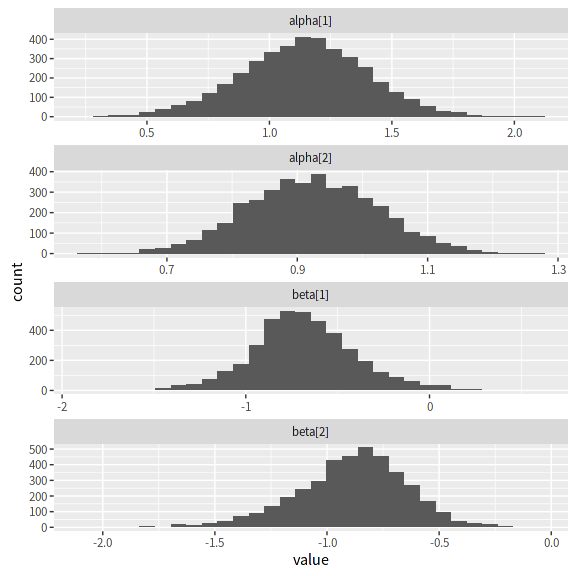Histogram (ggs_histogram()) The figure combines the values of all the chains. Although it is not specifically a convergence plot, it is useful for providing a quick look on the distribution of the values and the shape of the posterior distribution. ## Density plots ggs_density(S)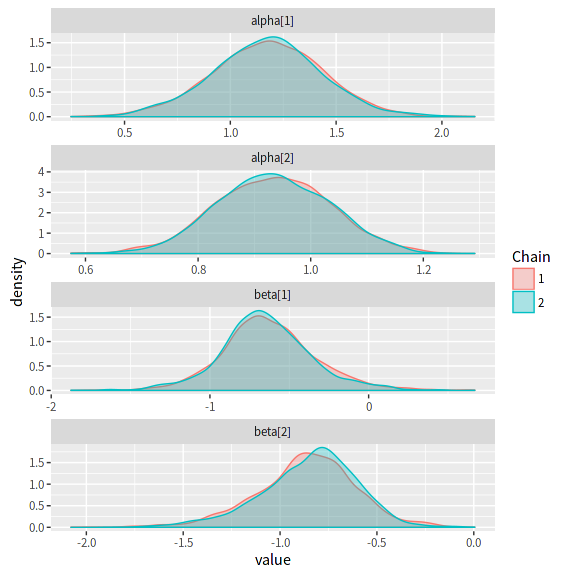Density plots (ggs_density()) ggs_density() produces overlapped density plots with different colors by chain, which allows comparing the target distribution by chains and whether each chain has converged in a similar space. ## Traceplots ggs_traceplot(S)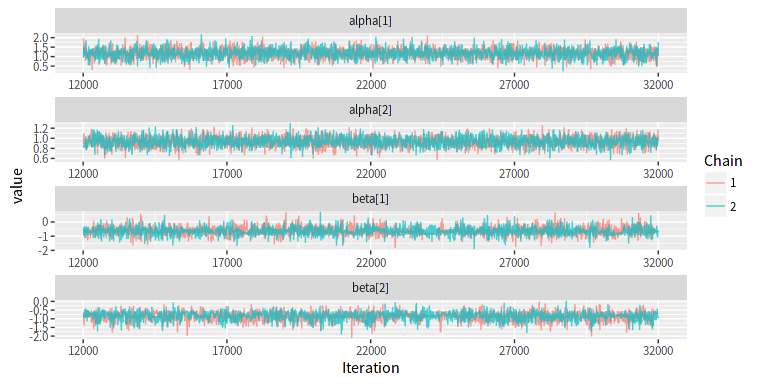Traceplots (ggs_traceplot()) A traceplot is an essential plot for assessing convergence and diagnosing chain problems. It basically shows the time series of the sampling process and the expected outcome is to produce “white noise”. Besides being a good tool to assess within-chain convergence, the fact that different colors are employed for each of the chains facilitates the comparison between chains. ## Running means ggs_running(S)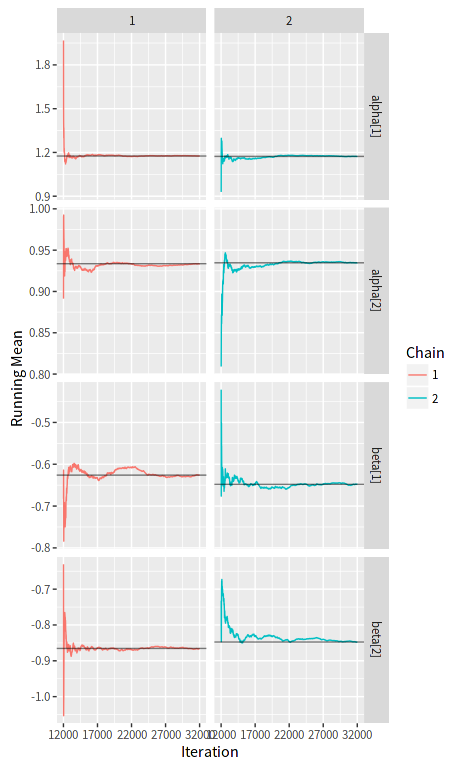Running means (ggs_running()) A time series of the running mean of the chain is obtained by ggs_running(), and allows to check whether the chain is slowly or quickly approaching its target distribution. A horizontal line with the mean of the chain facilitates the comparison. Using the same scale in the vertical axis also allows comparing convergence between chains. The expected output is a line that quickly approaches the overall mean, in addition to the fact that all chains are expected to have the same mean (which is easily assessed through the comparison of the horizontal lines). ## Comparison of the whole chain with the latest part ggs_compare_partial(S)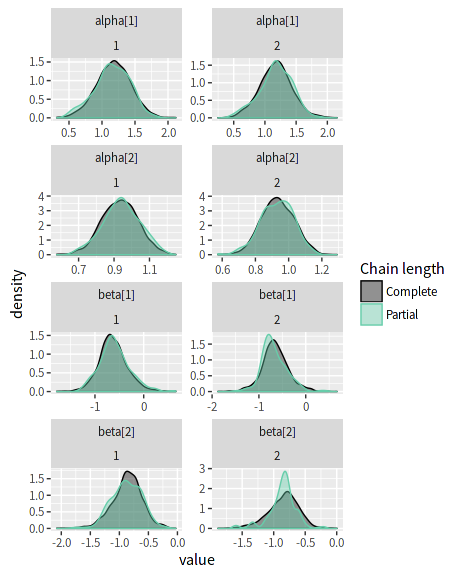Comparison of the whole chain with the latest part (ggs_compare_partial()) Based on the idea of overlapping densities, ggs_compare_partial() produces overlapped density plots that compare the last part of the chain (by default, the last 10 percent of the values, in green) with the whole chain (black). Ideally, the initial and final parts of the chain have to be sampling in the same target distribution, so the overlapped densities should be similar. Notice that the overlapping densities belong to the same chain, therefore the different columns of the plot refer to the different chains. ## Autocorrelation plots ggs_autocorrelation(S)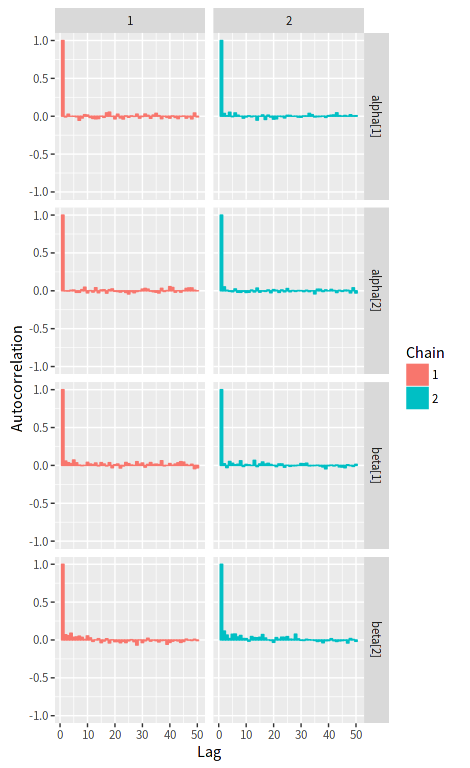Autocorrelation (ggs_autocorrelation()) The autocorrelation plot expectes a bar at one in the first lag, but no autocorrelation beyond it. While autocorrelation is not per se a signal of lack of convergence, it may indicate some misbehaviour of several chains or parameters, or indicate that a chain needs more time to converge. The easiest way to solve issues with autocorrelation is by thinning the chain. The thinning interval can be very easily extracted from the autocorrelation plot. By default, the autocorrelation axis is bounded between -1 and 1, so all subplots are comparable. The argument nLags allows to specify the number of lags to plot, which defaults to 50. ## Crosscorrelation plot ggs_crosscorrelation(S)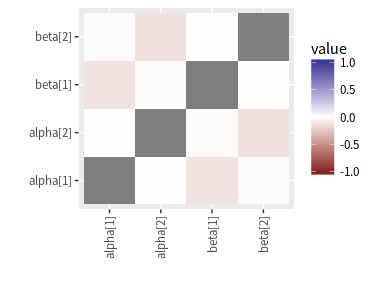Crosscorrelation (ggs_crosscorrelation()) In order to diagnose potential problems of convergence due to highly correlated parameters, ggs_crosscorrelation produces a tile plot with the correlations between all parameters. The argument absolute_scale allows to specify whether the scale must be between -1 and +1. The default is to use an absolute scale, which shows the crosscorrelation problems in overall perspective. But with cases where there is not a severe problem of crosscorrelation between parameters it may help to use relative scales in order to see the most problematic parameters. ## Potential Scale Reduction Factor ggs_Rhat(S) + xlab("R_hat")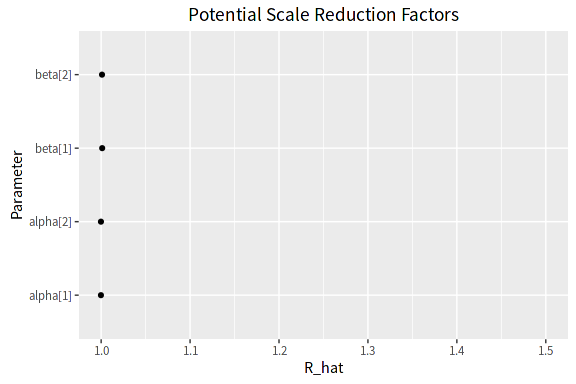Potential Scale Reduction Factor (ggs_Rhat()) The Potential Scale Reduction Factor ($$\hat{R}$$)(Gelman et al. 2003) relies on different chains for the same parameter, by comparing the between-chain variation with the within-chain variation. It is expected to be close to 1. ## Geweke Diagnostics ggs_geweke(S)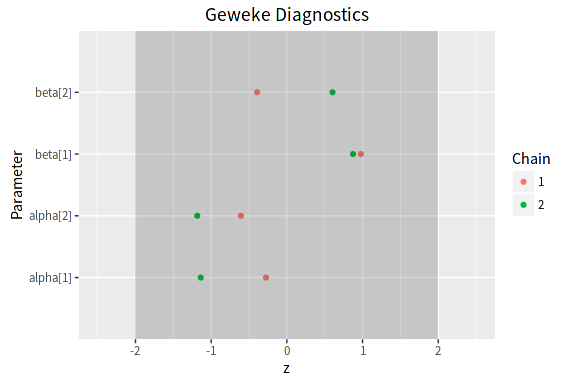Geweke diagnostic (ggs_geweke()) By contrast the Geweke z-score diagnostic (Geweke 1992) focuses on the comparison of the first part of the chain with its last part. It is in fact a frequentist comparison of means, and the expected outcome is to have 95 percent of the values between -2 and 2. By default, the area between -2 and 2 is shadowed for a quicker inspection of problematic chains. # Select a family of parametrs Suppose that the object with the results of a model contains several families of parameters (say, beta, theta, sigma) and that only one of the families you want to use the previous functions but only with one of the families. The argument family can then be used to indicate ggmcmc to use only certain parameters from the specified family. The following figure shows a density plot similar to a previous one, but with only the parameters that belong to the required family. In this case, all parameters that contain the character string sigma will be passed to ggs_density(). The character string provided to family can be any regular expression in R format. So in cases of having multidimensional arrays of parameters (say, theta[1,1] or theta[10, 5]), the elements of the first dimension being equal to 4 can be plotted using family="theta\$4,.\$". # Change parameter labels By default, ggs objects use the parameter names provided by the MCMC software. But it is possible to change it when treating the samples with the ggs() function using the argument par_labels. par_labels requires a data frame with at least two columns. One named Parameter with the corresponding names of the parameters that are to be changed and another named Label with the new parameter labels. P <- data.frame( Parameter=c("sigma.alpha", "sigma.beta", "sigma.y"), Label=c("Intercept (sd)", "Covariate (sd)", "Outcome (sd)")) ggs_density(ggs(radon$s.radon, par_labels=P, family="sigma"))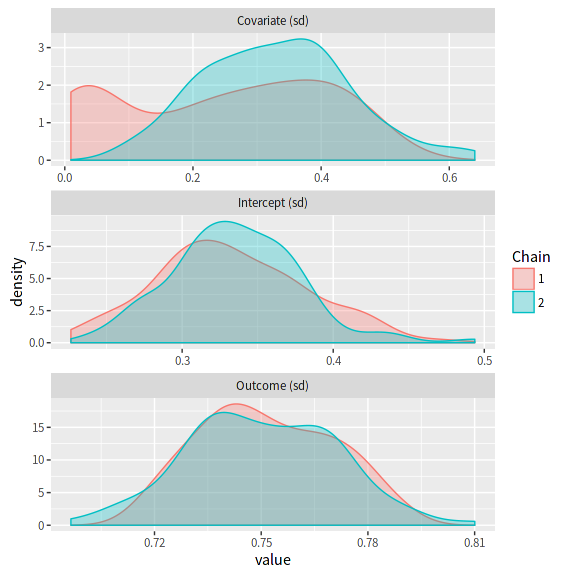Labels of the parameter names are changed by argument par_labels.

The combination of the arguments family and par_labels allows to have high control in terms of flexibility (which parameters are shown, using family) as well as in terms of substantial interpretation (the labels attached to each parameter, using par_labels). However, it must be noticed that selecting a family of parameters can be done either when converting the output of the MCMC software using the ggs() function, or inside the ggs_*() individual functions. But using the par_labels argument is only done through the ggs() call.

# Caterpillar plots

Caterpillar plots provide a very flexible graphical solution to interpret substantial results from Bayesian analysis, specially when dealing with lots of parameters, as is usually the case with hierarchical / multilevel models.

The simplest use is to get a sense of the distribution of the parameters, using their name as a label. The function produces caterpillar plots of the highest posterior densities of the parameters. By default, ggs_caterpillar() produces thick lines at the 90% highest posterior density (HPD) region and thin lines at the 95% HPD.

The following code creates a data set with the matches between the parameter names for the intercepts (alpha:alpha) and their substantial meaning (labels for counties) and then it passes this data frame as an argument to the ggs() function that converts the original samples in JAGS into a proper object ready for being used by all ggs_*() functions.

L.radon.intercepts <- data.frame(
Parameter=paste("alpha[", radon$counties$id.county, "]", sep=""),
Label=radon$counties$County)
head(L.radon.intercepts)
##   Parameter    Label
## 1  alpha   Aitkin
## 2  alpha    Anoka
## 3  alpha   Becker
## 4  alpha Beltrami
## 5  alpha   Benton
## 6  alpha Bigstone
S.full <- ggs(radon$s.radon, par_labels=L.radon.intercepts, family="^alpha") ggs_caterpillar(S.full)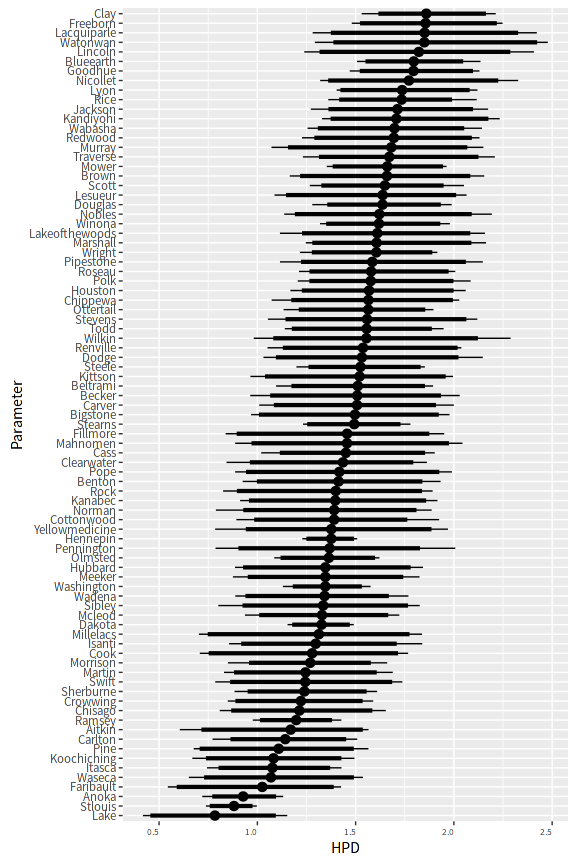Caterpillar plot (ggs_caterpillar()). ggs_caterpillar() can also be used to plot against continuous variables. The use of this feature may be very useful when plotting the varying slopes or intercepts of several groups in multilevel modelling against a continuous feature of such groups. This can be achieved by passing a data frame (X) with two columns. One column with the Parameter name and the other with its value. Notice that when used against a continuous variable it is more convenient to use vertical lines instead of the default horizontal lines. Z <- data.frame( Parameter=paste("alpha[", radon$counties$id.county, "]", sep=""), value=radon$counties$uranium) ggs_caterpillar(ggs(radon$s.radon, family="^alpha"), X=Z, horizontal=FALSE)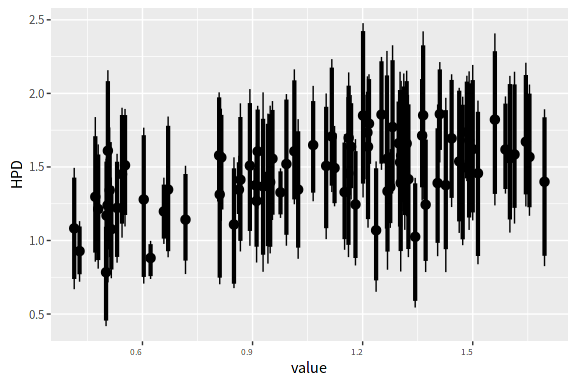Caterpillar plot against a continuous variable.

Another feature of caterpillar plots is the possibility to plot two different models, and be able to easily compare between them. A list of two ggs() objects must be provided.

The ci() function that calculates the credible intervals in the caterpillar plots can also be used outside the graphical display, generating a tbl_df suitable for other analysis.

ci(S)
## Source: local data frame [4 x 6]
##
##   Parameter        low        Low     median       High         high
##      <fctr>      <dbl>      <dbl>      <dbl>      <dbl>        <dbl>
## 1  alpha  0.6501846  0.7383305  1.1791994  1.6010471  1.679652649
## 2  alpha  0.7368340  0.7731033  0.9339059  1.1006331  1.130144748
## 3   beta -1.2057262 -1.0947502 -0.6543980 -0.1253985 -0.005177022
## 4   beta -1.4169397 -1.3104334 -0.8352727 -0.4804845 -0.404313590

# Posterior predictive checks and model fit

ggmcmc incorporates several functions to check model fit and perform posterior predictive checks. As to the current version, only outcomes in uni-dimensional vectors are allowed.

The outcomes can be continuous or binary, and different functions take care of them. The main input for the functions is also a ggs object, but in this case it must only contain the predicted values. $$\hat{y}_{i}$$ are the expected values in the context of the radon example. They can also be sampled and converted into a ggs object using the argument family.

In order to illustrate posterior predictive checks, samples from a faked dataset will be used. ggmcmc contains samples from a linear model with an intercept and a covariate (object s), where y.rep are posterior predictive samples from a dataset replicated from the original, but without the original outcome ($$y$$).

data(linear) # brings 's.y.rep', 'y' and 's'
S.y.rep <- ggs(s.y.rep)
y.observed <- y

## Continuous outcomes

For continuous outcomes, ggs_ppmean() (posterior predictive means) presents a histogram with the means of the posterior predictive values at each iteration, and a vertical line showing the location of the sample mean.

ggs_ppmean(S.y.rep, outcome=y.observed)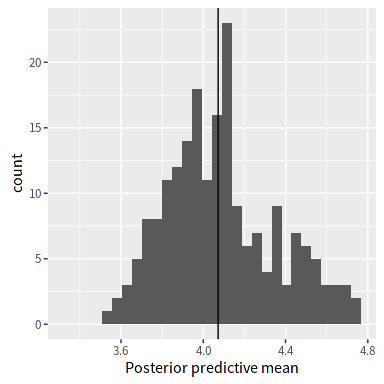This allows comparing the sample mean with the means of the posterior and check if there are substantial deviances.

ggs_ppsd() (posterior predictive standard deviations) presents the same idea as ggs_ppmean() but in this case using the posterior standard deviations.

ggs_ppsd(S.y.rep, outcome=y.observed)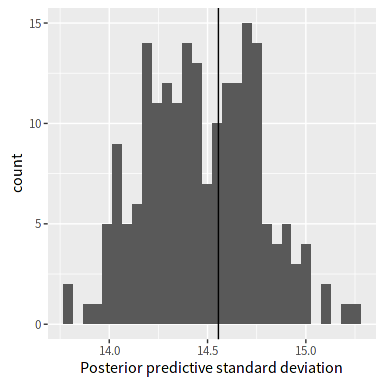Posterior predictive standard deviations against the sample standard deviation (ggs_ppsd()).

## Binary outcomes

In order to illustrate the functions suitable for binary outcomes a new dataset must be simulated, and samples of parameters from a simple logistic regression model must be obtained. ggmcmc also contains samples from such a model, in the dataset data(binary). Again, arrange the samples as a ggs object

data(binary)
S.binary <- ggs(s.binary, family="mu")

The ROC (receiver operating characteristic) plot presents a ROC curve.

ggs_rocplot(S.binary, outcome=y.binary)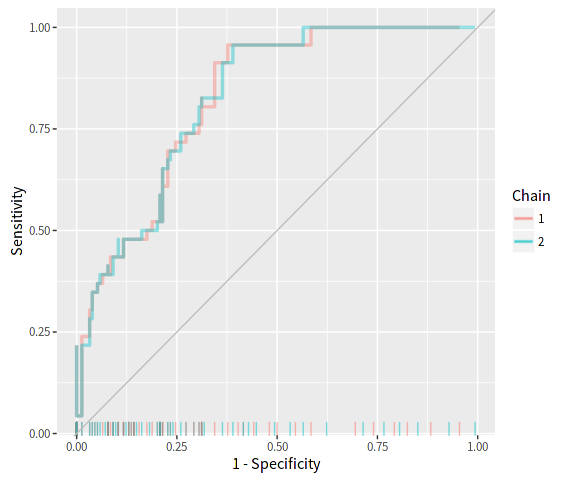The ggs_rocplot() is not fully Bayesian by default. This means that the predicted probabilities by observation are reduced to their median values across iterations from the beginning. Only information relative to the chain is kept. If a fully Bayesian version is desired, the argument fully_bayesian=TRUE has to be set up. But use it with care, because it is very demanding in terms of CPU and memory.

The separation plot (Greenhill, Ward, and Sacks 2011) is obtained by the ggs_separation function. The separation plot conveys information useful to assess goodness of fit of a model with binary outcomes (and also with ordered categories). The horizontal axis orders the values by increasing predicted probability. The observed successes (ones) have a darker color than observed failures (or zeros). Therefore, a perfect model would have the lighter colors in the right hand side, separated from darker colors. The black horizontal line represents the predicted probabilities of success for each of the observations, which allows to easily evaluate whether there is a strong or a weak separation between successes and failures predicted by the model. Lastly, a triangle on the lower side marks the expected number of total successes (events) predicted by the model.

ggs_separation(S.binary, outcome=y.binary)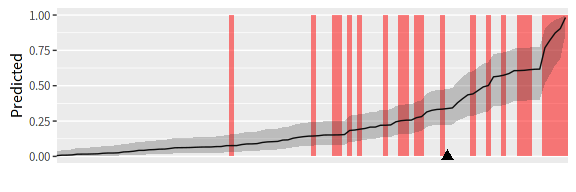Separation plot.

The arguments show_labels (FALSE by default) and uncertainty_band (TRUE by default) allow to control, respectively, whether the Parameter names will be displayed and whether the uncertainty of the predicted values will be shown.

ggs_separation(S.binary, outcome=y.binary,
show_labels = TRUE, uncertainty_band = FALSE)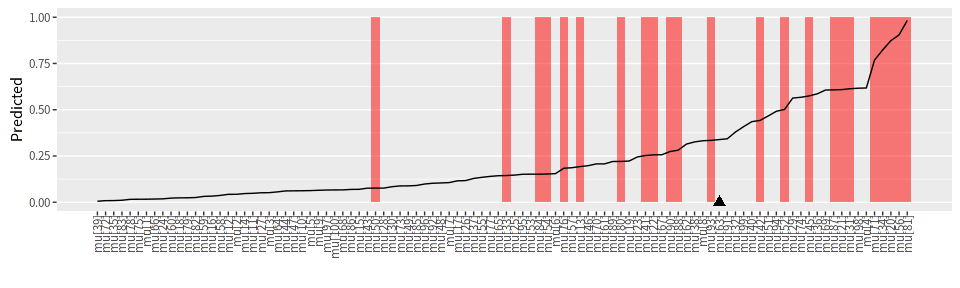Separation plot with parameter labels and without uncertainty band on the predicted values.

The argument minimalist (FALSE by default) allows get a minimal Tufte style inline version of the separation plot.

ggs_separation(S.binary, outcome=y.binary, minimalist = TRUE)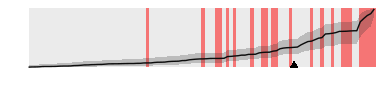Separation plot (minimalist version).

# Paired plots

The package ggally provides an easy way to extend ggmcmc through the ggs_pairs() function, which by defaults shows the scatterplots of the pairs of parameters in the ggs object, the densities and the crosscorrelations in a single layout. In the following example, the lower argument to ggs_pairs() is passed to ggpairs() as a list so that the figures in the lower quadrant are not scatterplots, but contours instead.

ggs_pairs(S, lower = list(continuous = "density"))